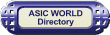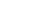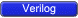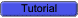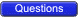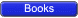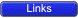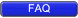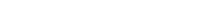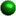Logic Circuit Modeling From what we have learnt in digital design, we know that there could be only two types of digital circuits. One is combinational circuits and the second is sequential circuits. There are very few rules that need to be followed to get good synthesis output and avoid surprises.Combinational Circuit Modeling using assign Combinational circuits modeling in Verilog can be done using assign and always blocks. Writing simple combinational circuits in Verilog using assign statements is very straightforward, like in the example belowassign y = (a&b) | (c^d);Tri-state buffer``` 1 module tri_buf (a,b,enable); 2 input a; 3 output b; 4 input enable; 5 wire a,enable; 6 wire b; 7 8 assign b = (enable) ? a : 1'bz; 9 10 endmodule ``` You could download file tri_buf.v hereMux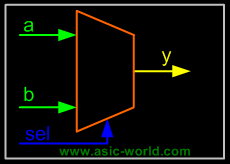``` 1 module mux_21 (a,b,sel,y); 2 input a, b; 3 output y; 4 input sel; 5 wire y; 6 7 assign y = (sel) ? b : a; 8 9 endmodule ``` You could download file mux_21.v hereSimple Concatenation``` 1 module bus_con (a,b); 2 input [3:0] a, b; 3 output [7:0] y; 4 wire [7:0] y; 5 6 assign y = {a,b}; 7 8 endmodule ``` You could download file bus_con.v here1 bit adder with carry``` 1 module addbit ( 2 a , // first input 3 b , // Second input 4 ci , // Carry input 5 sum , // sum output 6 co // carry output 7 ); 8 //Input declaration 9 input a; 10 input b; 11 input ci; 12 //Ouput declaration 13 output sum; 14 output co; 15 //Port Data types 16 wire a; 17 wire b; 18 wire ci; 19 wire sum; 20 wire co; 21 //Code starts here 22 assign {co,sum} = a + b + ci; 23 24 endmodule // End of Module addbit ``` You could download file addbit.v hereMultiply by 2``` 1 module muliply (a,product); 2 input [3:0] a; 3 output [4:0] product; 4 wire [4:0] product; 5 6 assign product = a << 1; 7 8 endmodule ``` You could download file multiply.v here3 is to 8 decoder``` 1 module decoder (in,out); 2 input [2:0] in; 3 output [7:0] out; 4 wire [7:0] out; 5 assign out = (in == 3'b000 ) ? 8'b0000_0001 : 6 (in == 3'b001 ) ? 8'b0000_0010 : 7 (in == 3'b010 ) ? 8'b0000_0100 : 8 (in == 3'b011 ) ? 8'b0000_1000 : 9 (in == 3'b100 ) ? 8'b0001_0000 : 10 (in == 3'b101 ) ? 8'b0010_0000 : 11 (in == 3'b110 ) ? 8'b0100_0000 : 12 (in == 3'b111 ) ? 8'b1000_0000 : 8'h00; 13 14 endmodule ``` You could download file decoder.v hereCombinational Circuit Modeling using always While modeling using always statements, there is the chance of getting a latch after synthesis if care is not taken. (No one seems to like latches in design, though they are faster, and take lesser transistor. This is due to the fact that timing analysis tools always have problems with latches; glitch at enable pin of latch is another problem).One simple way to eliminate the latch with always statement is to always drive 0 to the LHS variable in the beginning of always code as shown in the code below.3 is to 8 decoder using always``` 1 module decoder_always (in,out); 2 input [2:0] in; 3 output [7:0] out; 4 reg [7:0] out; 5 6 always @ (in) 7 begin 8 out = 0; 9 case (in) 10 3'b001 : out = 8'b0000_0001; 11 3'b010 : out = 8'b0000_0010; 12 3'b011 : out = 8'b0000_0100; 13 3'b100 : out = 8'b0000_1000; 14 3'b101 : out = 8'b0001_0000; 15 3'b110 : out = 8'b0100_0000; 16 3'b111 : out = 8'b1000_0000; 17 endcase 18 end 19 20 endmodule ``` You could download file decoder_always.v hereSequential Circuit Modeling Sequential logic circuits are modeled using edge sensitive elements in the sensitive list of always blocks. Sequential logic can be modeled only using always blocks. Normally we use nonblocking assignments for sequential circuits.Simple Flip-Flop``` 1 module flif_flop (clk,reset, q, d); 2 input clk, reset, d; 3 output q; 4 reg q; 5 6 always @ (posedge clk ) 7 begin 8 if (reset == 1) begin 9 q <= 0; 10 end else begin 11 q <= d; 12 end 13 end 14 15 endmodule ``` You could download file flip_flop.v hereVerilog Coding Style If you look at the code above, you will see that I have imposed a coding style that looks cool. Every company has got its own coding guidelines and tools like linters to check for this coding guidelines. Below is a small list of guidelines.Use meaningful names for signals and variables Don't mix level and edge sensitive elements in the same always block Avoid mixing positive and negative edge-triggered flip-flops Use parentheses to optimize logic structure Use continuous assign statements for simple combo logic Use nonblocking for sequential and blocking for combo logic Don't mix blocking and nonblocking assignments in the same always block (even if Design compiler supports them!!). Be careful with multiple assignments to the same variable Define if-else or case statements explicitlyNote : Suggest if you want more details.